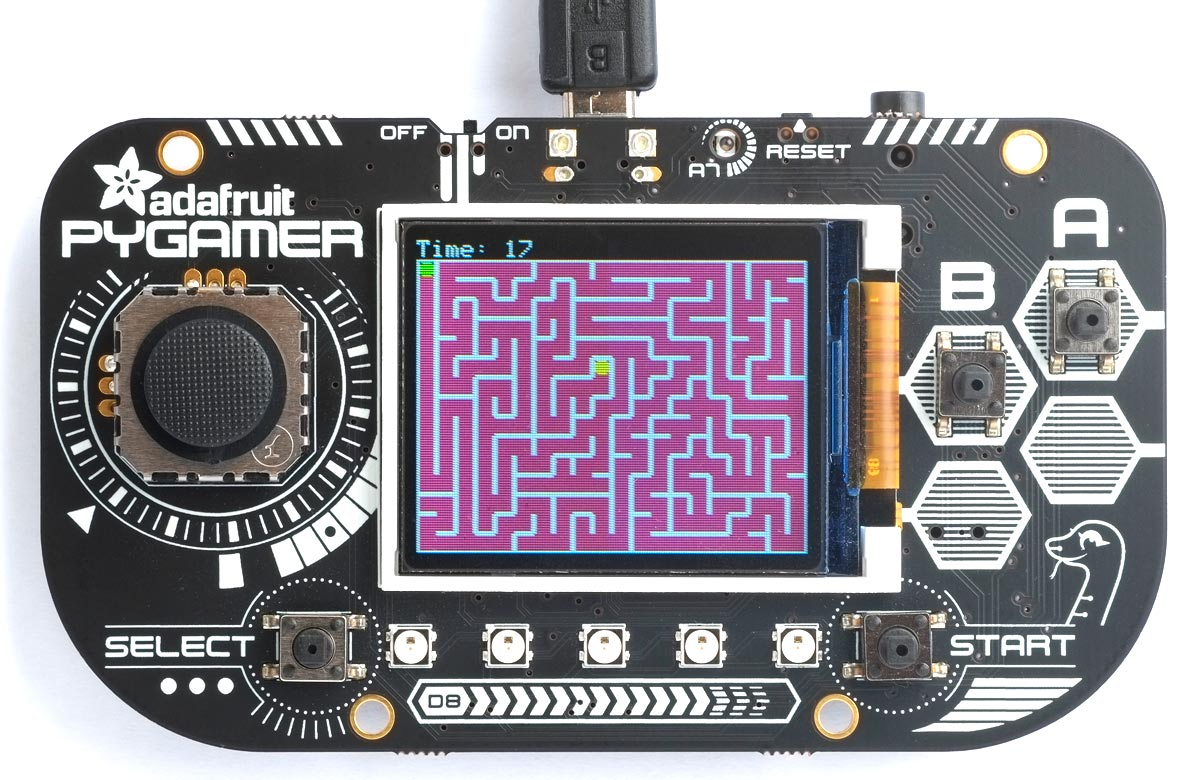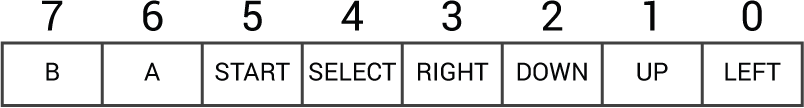## Simple maze game

Here's another arcade-style game written in uLisp, suitable for the Adafruit PyBadge or PyGamer. It's a maze game in which you have to use the joystick to move a ball through a randomly-generated maze to reach the goal in the shortest possible time:This game first appeared on the uLisp Forum.

9th February 2023: Updated with clearer instructions of how to install and run the game.

### Running the maze game

To run the game you need an Adafruit PyGamer , which is a microcontroller board based on the ATSAMD51 with GameBoy-style control buttons, and a 1.8" 160x128 colour TFT display. The game will also work on the similar Adafruit PyBadge  and PyBadge LC .

• Include the graphics extensions before installing it by enabling the option:
`#define gfxsupport`
• Open the Serial Monitor in the Arduino IDE to display the uLisp prompt.
• Display the source of the Maze Game here: PyGamer Maze game.
• Select the text of the maze game, copy it, paste it into the Arduino IDE Serial Monitor input field, and press Return.
• Run the game by typing the following command into the Arduino IDE Serial Monitor input field, followed by Return:
`(play)`
• Use the PyGamer joystick or PyBadge keypad to navigate the ball through the maze.

### How it works

There are many alternative methods for drawing a random maze; the one I chose is called recursive backtracking because it's one of the simplest, and produces good mazes.

The maze is drawn on a grid of 20 x 15 cells, defined by mwidth and mheight. The cell spacing is 8 pixels, defined by gap, and the origin of the maze is defined by (mx, my), to leave the top row of 8 pixels clear for displaying the score. The cells are 6 pixels square, and the walls are 1 pixel thick. These parameters are defined by the following constants:

```; Maze parameters
(defvar mwidth 20)
(defvar mheight 15)
(defvar gap 8)
(defvar mx 0)
(defvar my 8)
(defvar cellsize 6)
(defvar wall 1)```

The maze is constructed using a two-dimensional global array grid to represent the cells:

`(setq grid (make-array (list mwidth mheight) :initial-element 0))`

Each element of the array contains a four-bit value to specify which of the four walls are present around the cell. During the construction of the maze a cell that hasn't already been visited has a zero value.

The functions dx and dy help calculate the coordinates of a cell in each of the four compass directions:

```; Directions W N S E
(defun dx (dir) (nth dir '(-1  0  0  1)))
(defun dy (dir) (nth dir '( 0 -1  1  0)))```

The directions are arranged so that we can easily calculate the opposite of a direction:

`(defun opposite (dir) (- 3 dir))`

#### Initialising the maze

First we initialise the maze by drawing a rectangle filled with the maze colour, mazecol. Initially the starting cell, corresponding to array element (0, 0), is cleared to the board colour, boardcol:

```(defun init-maze ()
(fill-rect mx my (* gap mwidth) (* gap mheight) mazecol)
(fill-rect (+ mx wall) (+ my wall) cellsize cellsize boardcol)
(setq grid (make-array (list mwidth mheight) :initial-element 0))
(setq maxdepth 0)
(create-maze 0 0 0))```

Then we call create-maze to create the maze in the array grid.

#### Creating the maze

The maze is actually constructed by the recursive routine create-maze, which is called with a starting cell (ax, ay):

```(defun create-maze (ax ay depth)
(when (> depth maxdepth) (setf gx ax gy ay maxdepth depth))
(let ((r (random 4)))
(dotimes (d 4)
(let* ((dir (mod (+ d r) 4))
(bx (+ ax (dx dir)))
(by (+ ay (dy dir))))
(when (and (< -1 bx mwidth) (< -1 by mheight) (zerop (aref grid bx by)))
(setf (aref grid bx by) (ash 1 (opposite dir)))
(setf (aref grid ax ay) (logior (aref grid ax ay) (ash 1 dir)))
(create-maze bx by (incf depth)))))))```

From the starting square (ax, ay) it extends the maze one square in a random direction, W, N, S, or E, to a second square (bx, by). If this a valid cell on the grid, and it hasn't already been visited, the following steps are performed:

• A bit corresponding to the wall is set in the grid array element (bx, by).
• A bit corresponding to the wall is set in the grid array element (ax, ay).
• The routine create-maze is called recursively with the coordinates of the second cell (bx, by) to continue extending the maze.

As the maze is constructed the routine sets (gx, gy) to the coordinates of the cell at the maximum depth of recursion. This is a simple way of finding a good goal for solving the maze.

#### Drawing the maze

The routine draw-maze draws the maze from the array grid. For each cell it draws whichever of the four walls should be present:

```(defun draw-maze ()
(dotimes (x mwidth)
(dotimes (y mheight)
(let* ((cell (aref grid x y))
(cx (+ mx (* x 8)))
(cy (+ my (* y 8)))
(dx (+ cx gap -1))
(dy (+ cy gap -1)))
(unless (logbitp 0 cell) (draw-line cx cy cx dy))
(unless (logbitp 1 cell) (draw-line cx cy dx cy))
(unless (logbitp 2 cell) (draw-line cx dy dx dy))
(unless (logbitp 3 cell) (draw-line dx cy dx dy))))))```

The following routines reads the PyGamer joystick, and converts the joystick values to directions:

```(defun joystick ()
(let ((x 0) (x0 536) (y 0) (y0 523))
(list (- (truncate x 3) x0) (- (truncate y 3) y0))))

(let ((buttons 0) (clock 48) (data 49) (latch 50))
(pinmode clock t) (digitalWrite clock 0)
(pinmode latch t) (digitalWrite latch 1)
(pinmode data nil)
(digitalwrite latch 0) (digitalWrite latch 1)
(dotimes (b 8 buttons)
(setq
buttons
(logior (ash buttons 1) (if (digitalread data) 1 0)))
(digitalwrite clock 1) (digitalwrite clock 0))
(let* ((j (joystick))
(x (first j))
(y (second j)))
(logior
buttons
(cond ((< x -350) 1) ((< y -350) 2) ((> y 350) 4) ((> x 350) 8) (t 0))))))```

Each bit in the result from (readgamerbuttons) corresponds to one of the eight possible buttons:This is compatible with the result returned by the PyBadge direction buttons.

#### The main program

The main program play builds the maze, and then updates the display as you use the joystick to mave the ball through the maze. It uses sprite to draw a round rectangle to represent the ball and goal:

```(defun sprite (x y col)
(fill-round-rect (+ (* 8 x) mx 1) (+ (* 8 y) my 1) cellsize cellsize 2 col))```

and display to display a line of text on the top line of the display:

```(defun display (time)
(with-gfx (str)
(fill-rect 0 0 160 8 0)
(set-cursor 0 0)
(format str "Time: 17" time)))```

Here's the main play routine:

```(defun play ()
(let ((direction 1) (time 0) (score 0)
(x 0) (y 0) (start (millis)))
(init-maze)
(draw-maze)
(sprite x y green)
(sprite gx gy gold)
(loop
(let ((now (truncate (- (millis) start) 1000)))
(when (> now time)
(setq time now)
(display time)))
(let ((r (case (readgamerbuttons) (1 0) (2 1) (4 2) (8 3) (t nil))))
(when r
(setq direction r)
; Are we allowed to go that way?
(when (logbitp direction (aref grid x y))
; Undraw old position and draw new one
(sprite x y mazecol)
(incf x (dx direction))
(incf y (dy direction))
; At goal?
(when (and (= x gx) (= y gy)) (loop))
(sprite x y green))))
(delay 100))))```

### Resources

Here's the whole game in a single file: PyGamer Maze game.